# Digital Parallel and Serial Converters Information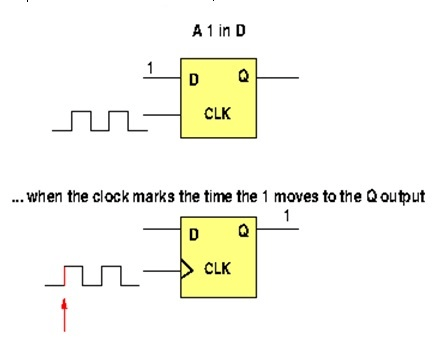Digital parallel and serial converters adapt data transmitted in a parallel port to a serial communication format, or adapt serial port data to parallel communication format.

Data converters are needed because not all devices in a system work entirely as parallel-only or serial-only components. For instance, the communication inside a computer uses parallel buses that transmit parallel data from component to component. However communication among external devices take place using serial data. A network of computer receives data from the internet using serial communication. When the data arrives at a particular network node, the communication switches to parallel data transmission.

Converters are designed using D flip-flops (D latches work as well). A D flip-flop transfers one bit from its input to its output every time the clock shifts from low to high (if the device is a positive-edge triggered flip-flop), or from high to low (if the device is a negative-edge triggered flip-flop.) If, for instance, there is high (logic 1) at the input D of the flip-flop, then when the clock edge goes from low to high the logic 1 in the input is transferred to the output terminal, the Q pin. This situation is depicted in the figure at right.

If another D flip-flop is connected to the output terminal of the first, then we can transfer two bits of data with two ticks of the clock. The following figures depict this situation. The bits 0 and 1 are at the input of the first flip-flop. At the first tick of the clock the first bit is transferred to the output of the first flip-flop, which becomes the input of the second. Now there is one bit (0) at the input of the first and a bit (1) at the input of the second flip-flop. At the second tick of the clock there is a transfer that puts one bit (0) at the input of the second flip-flop and a bit (1) at its output. This process is repeated if there are more flip-flops connected in series. In general, transferring n bits requires n flip-flops.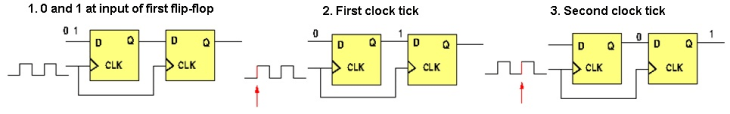##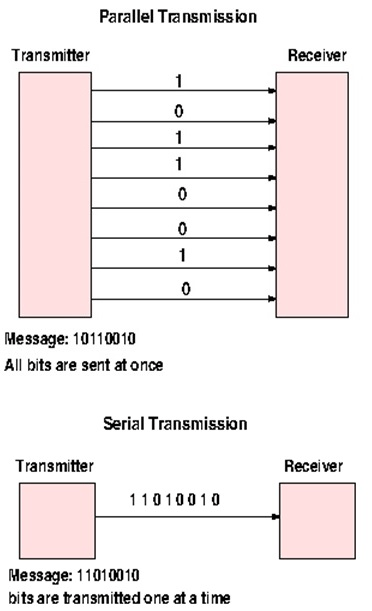Transmissions

There are the two basic types of transmission modes available in digital systems, serial and parallel transmission.

Parallel Transmission

Parallel transmission uses one communication line for each bit of the message. For example, to send a message of eight bits, eight wires are required from transmitter to receiver. The ensemble of the eight wires is called a data bus. Parallel transmission is normally used when the physical distance between the transmitter and the receiver is short, because is difficult to maintain bit synchronization in long transmission. Typically parallel transmission is preferred within a device; communication pathways between the CPU and memory of a computer are parallel. There are several protocols used in parallel data transmission throughout the industry, including parallel PCI, ISA, ATA, and SCSI. The accompanying figure depicts the transmission for an eight-bit message using a parallel bus.

Serial Transmission

Each bit of the message is sent to the receiver one bit at a time using one communication line. Most communication is carried out using serial data. Serial communication requires less wires and less complex electronics, but to compare parallel data it must transmit at a faster rate. Examples of serial data protocols include Internet, USB, SPI, I2C , Firewire and others.

## Conversions

Serial to Parallel Conversion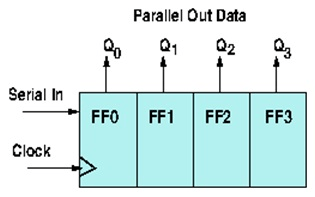To convert serial data to parallel data a set of D flip-flops is needed. The number of flip-flops is exactly the size of the serial data to be transmitted. For example, to transmit four-bit serial stream four flip-flops a required. A schematic of a four-bit converter is depicted.

The serial data is delivered at the input of the first flip-flop, and bits are successfully transferred to the next flip-flop on the rising (or falling) edge of the clock. The next figure shows an actual circuit for a four-bit converter, where four bits (0, 0, 0, and 1) are stored at the input of the first flip-flop.

With the first rising edge (i.e. tick) of the clock, the first bit (1 in this case) is transferred to the input of the second flip-flop. Successive ticks moves the bits to the next flip-flop, until all four bits are stored at the output of each flip-flop. In this figure we have not shown all the circuitry of an actual converter. The converter does not release the parallel set of bits until all the bits (four in this case) are transferred, and each one is stored at the output (Q) of a corresponding flip-flop. Once all the outputs are filled, the converter releases all the bits at once. For this process to happen, the converter is disabled (by means of one or more control lines) during the transfer process and enabled once all the bits are at the output bus. This is summarized by stating that the conversion is carried out in three stages:

1. Disable the output bus. The converter can't send output data.
2. Load all the bits into the outputs of the flip-flops by moving them one bit at a time using the clock.
3. Once all the bits are loaded (all the flip-flops have one bit stored in the Q pin), then enable the bus operation. The four bits are sent at once.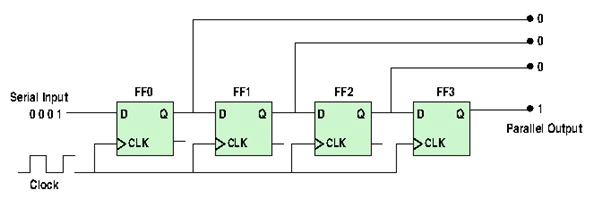Parallel to Serial Conversion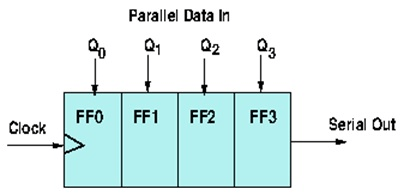In this converter all parallel data is loaded (stored) simultaneously into the D-type flip-flops. Once this is achieved, with the help of the clock, data is shifted one bit a a time from the last flip-flop. This two-step process is schematically illustrated in the accompanying figure.

In an actual converter, more circuitry is needed. Simply, the parallel data is multiplexed in order to convert it into serial data. The multiplexer will force the parallel data to be shifted one bit at a time through the last (most significant bit) flip-flop. The following figure is the diagram of a four bit converter. There are four flip-flops and three multiplexers. Each flip-flop is the output of a multiplexer, with the exception of the first flip-flop, which will represent the least significant bit (LSB) of the output serial data. Each multiplexer has two inputs (known as a 2 x 1 mux) and one output. The inputs are one bit of the parallel data and one input from the previous flip-flop.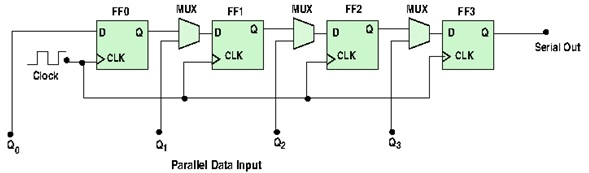## Specifications

• Clock speed. Normally given in Hz, it is the speed at which the data is shifted inside the converter
• The size of the converter. This is the number of bits the converter can handle
• Power. The total power needed to operate the device (current or voltage, or both)

#### Image credits:

Engineering360